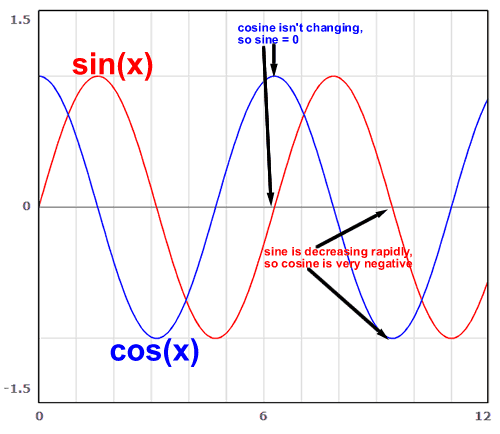# Compute Derivatives for Trig Functions

While you may know how to take the derivative of a polynomial, what happens when you need to take the derivative of a trig function? As a starting point, what is the derivative of a sine function?

$$\frac{d}{dx}\sin(x) = ?$$

Luckily, the derivatives of trig functions are simple -- they're other trig functions! For example, the derivative of sine is just cosine:

$$\frac{d}{dx}\sin(x) = \cos(x)$$

The chain rule still applies here when working with more complex functions:

$$\frac{d}{dx}\sin(3x^2) = 6x*\cos(3x^2)$$

The rest of the trig functions are also straightforward once you learn them, but they aren't QUITE as easy as the first two.

### Derivatives of Trigonometry Functions

$$\sin'(x) = \cos(x)$$ $$\cos'(x) = -\sin(x)$$ $$\tan'(x) = \sec^2(x)$$ $$\sec'(x) = \sec(x)\tan(x)$$ $$\cot'(x) = -\csc^2(x)$$ $$\csc'(x) = -\csc(x)\cot(x)$$

### How did we calculate these functions?

The characteristic trigonometric identity to recall in calculus is this: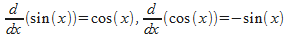It says that the derivative of sine is cosine, and the derivative of cosine is negative sine.

From these we may derive the rest of the derivatives, via the Quotient and Product rules. See if you can follow along as we derive them!

### Derivative of Secant

Remember that the secant is the inverse of cosine -- it's 1/cos(x). Rewrite it as such, and find the derivative: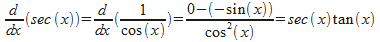All we did was think of 1/cos(x) as cos(x) to the -1 power. Finally we simplified a bit by converting sin/cos to tan and the remaining 1/cos term to sec.

### Derivative of Cosecant

Just like secant, except with sine instead of cosine. That's because cosecant is 1/sin!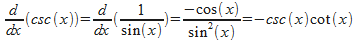### Derivative of Tangent

For tangent, let's rewrite tangent as sin*sec. Remember that tangent is sin/cos, which is the same as sin*sec. It's often easier to differentiate a product, so we'll use that version and substitute the derivative of sec from above.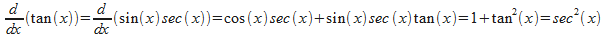### Derivative of Cotangent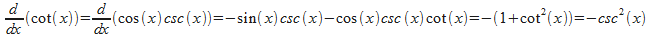### To sum up: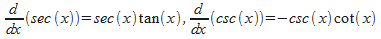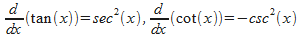So, that's how we mathematically derive the derivative of each trig function. Let's step back though a visually demonstrate the relationship between sine and cosine, and the neat fact that they are each other's derivatives (plus a negative sign when appropriate). Take a look at this graphic for an illustration of what this means. At the first point (around x=2*pi), the cosine isn't changing. You can see that the sine is 0, and since negative sine is the rate of change of cosine, cosine would be changing at a rate of -0.

At the second point I've illustrated (x=3*pi), you can see that the sine is decreasing rapidly. This makes sense because the cosine is negative. Since cosine is the rate of change of sine, a negative cosine means the sine is decreasing.